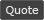ESOUI - View Single Post - Is there a global public function to safely update the map?
 View Single Post
 01/08/19, 03:47 AM #6 Hydra9268 Join Date: May 2018 Posts: 30 I see what the problem is. Zygor isn't using the LibGPS. They created their own metatable overload! Which means ZO_WorldMap_RefreshCustomPinsOfType(_G[pinType]) doesn't work. WTF Zygor? Oh, and all the crap below ESOdot isn't used. Looks like they were doing something with marching ants? lua Code: `Pointer.Icons = {    default =   "ESOdot",    ESOdot =    { icon=ZGV.DIR.."/Arrows/Stealth/arrow-error.dds", size=40, minisize=25, rotates=false, edgeicon=ZGV.DIR.."\\Skins\\minimaparrow-green-edge", edgesize=60, spinner=true, onminimap=always },    greendot =  { icon=ZGV.DIR.."\\Skins\\minimaparrow-green-dot", size=40, minisize=25, rotates=false, edgeicon=ZGV.DIR.."\\Skins\\minimaparrow-green-edge", edgesize=60, spinner=true, onminimap=always },    graydot =   { icon=ZGV.DIR.."\\Skins\\minimaparrow-green-dot", size=40, minisize=25, rotates=false, edgeicon=ZGV.DIR.."\\Skins\\minimaparrow-green-edge", edgesize=60, spinner=true, desat=1, onminimap=always },    arrow =     { icon=ZGV.DIR.."\\Skins\\minimaparrow-path", size=70, minisize=60, rotates=true, edgeicon=ZGV.DIR.."\\Skins\\minimaparrow-path", edgesize=50 },    ant =       { icon=ZGV.DIR.."\\Skins\\minimaparrow-ant", alpha=0.8, size=30, minisize=25, rotates=false, edgeicon=nil, edgesize=1 },    ant_g =     { icon=ZGV.DIR.."\\Skins\\minimaparrow-ant", r=0.4, g=1, b=0, alpha=0.8, size=30, minisize=25, rotates=false, edgeicon=nil, edgesize=1 },    ant_b =     { icon=ZGV.DIR.."\\Skins\\minimaparrow-ant", r=0, g=0.7, b=1, alpha=0.8, size=30, minisize=25, rotates=false, edgeicon=nil, edgesize=1 },    ant_p =     { icon=ZGV.DIR.."\\Skins\\minimaparrow-ant", r=0.8, g=0.3, b=1, alpha=0.8, size=30, minisize=25, rotates=false, edgeicon=nil, edgesize=1 },    ant_y =     { icon=ZGV.DIR.."\\Skins\\minimaparrow-ant", r=1, g=0.8, b=0, alpha=0.8, size=30, minisize=25, rotates=false, edgeicon=nil, edgesize=1 },    none =      { icon="", alpha=0.0, size=1, minisize=1, rotates=false, edgeicon=nil, edgesize=1 },     ant_g_default = { r=0.4, g=1, b=0, alpha=0.8 },    ant_b_default = { r=0, g=0.7, b=1, alpha=0.8 },    ant_p_default = { r=0.8, g=0.3, b=1, alpha=0.8 },    ant_y_default = { r=1, g=0.8, b=0, alpha=0.8 },    ant_default = { r=1, g=1, b=1, alpha=0.8 },} setmetatable(Pointer.Icons,{__index=function(k) return Pointer.Icons[Pointer.Icons.default] end})for k,v in pairs(Pointer.Icons) do if type(v)=="table" then __CLASS[v]="PointerIcon_"..k end end` And here's how they get the pin. lua Code: `function Pointer:GetMapMarker (m,f,x,y,data)    if not m and not f then        m,f = self:GetMapTex(),0    end     if not m or not f or not x or not y then        Pointer:Debug("GetMapMarker bailing out; map=%s/%d %.2f %.2f",m,f or -1,x or -99,y or -99)        return    end     local waypoint = self:GetUnusedMarker()    waypoint.m = m    waypoint.f = f    waypoint.x = x    waypoint.y = y     ZGV.Utils.table_join(waypoint,data)    return waypointend` The code is gross! This whole time I thought they were using LibGPS. It looks like they loaded the library into the txt file and never bothered to use it. Well, I guess that's good news. It means I can rip out all their bull**** and move to LibsGPS and hopefully make this Addon more friendly with other Addons like Bandit's mini-map. I'm not at that level of LUA scripting but I need to give it go out of necessity. Hopefully, it's not too too complicated with one pin. There's also this awesome HOW-TO guide by Garkin. I hope it is still relevant in 2018. If you know of a updated guide or a better guide please let me know. I'll take all the guidance I can get.